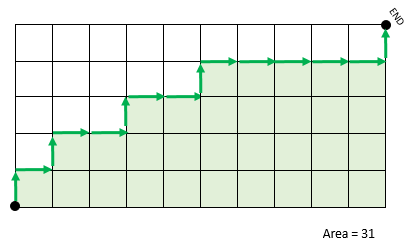# That Creepy Extra Area Under the Stairs

Probability Level 2

Start in the lower left corner of a 5x10 unit grid. You may only move to the right and upward along the edges to get to the top right corner. The area of a path between the corners is the amount of area beneath the line in square units. For example:On a 5x10 grid, how many different paths from the lower left corner to the upper right corner have an area congruent to 1 mod 5?

Bonus: Choose any prime $p$ and positive integers $i, $j$ and $k$ and generalize this solution to the number of paths with an area congruent to $i$ mod $p$ on a $jp$ by $kp$ grid.

×

Problem Loading...

Note Loading...

Set Loading...